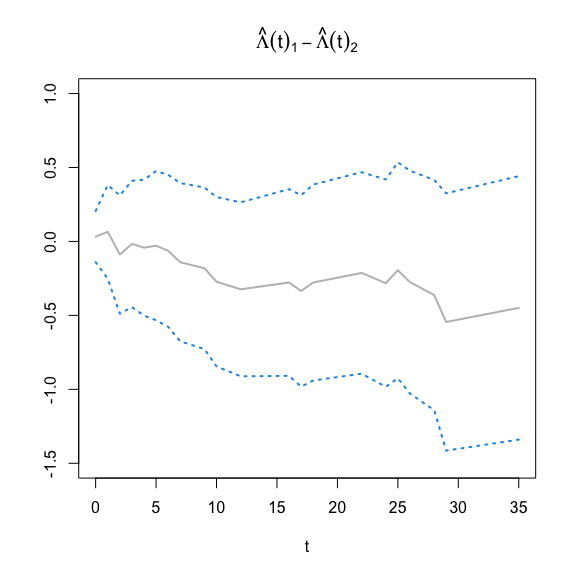# opt.ci

### Introduction to optband

#### 2023-03-30

Classical simultaneous confidence bands for survival functions (Hall and Wellner, 1980; Nair, 1984) are derived from transformations of the convergence to a Weiner process with a strictly-increasing variance function These transformations are often motivated by factors such as:

• Time intervals for which the analyst prefers to be thinner or wider
• Tractability of computing critical values of the asymptotic distribution in attaining the prescribed (nominal) coverage level

This package instead approaches the problem purely from an optimization perspective: given a certain coverage level, obtain bands such that the area between is minimized. While an exact solution cannot be obtained in closed form, optband provides an approximate solution based off local time arguments for both the survival and cumulative-hazard functions, generalizing the results specified by Kendall et al. (2007).

## Usage

opt.ci takes a survfit object from the survival package with the desired $$1-\alpha$$ coverage level, function of interest (either 'surv' for the survival function or 'cumhaz' for the cumulative-hazard function), and optional upper or lower bounds for data truncation. Defaults are $$\alpha=0.05$$, fun = 'surv', tl = NA, tu = NA.

## Example

First, let’s generate some survival data using the stats package and estimate the Kaplan-Meier curve:

set.seed(1990)
N = 200
x1 <- stats::rweibull(N, 1, 1)
x2 <- stats::rweibull(N, 2, 1)
d <- x1 < x2
x <- pmin(x1,x2)
mydata = data.frame(stop = x, event = d)
S = survival::survfit(Surv(x, d) ~ 1, type="kaplan-meier")

Now we can estimate the optimized confidence bands and plot the curve:

opt_S <- optband::opt.ci(S, conf.level = 0.95, fun = "surv", tl = NA, tu = NA)
plot(opt_S, xlab="time", ylab="KM", mark.time=FALSE)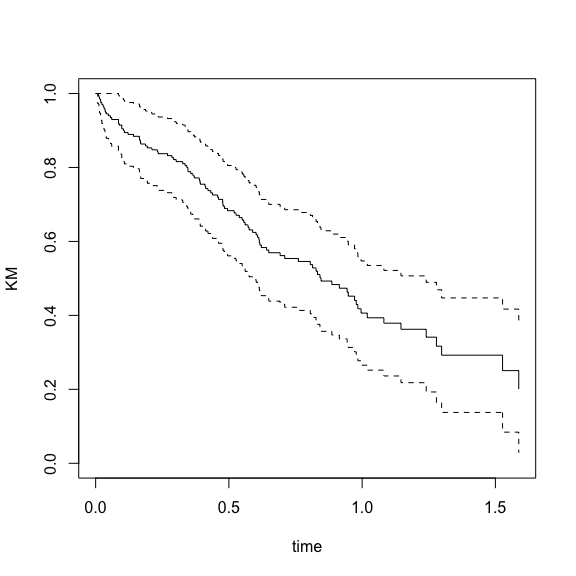And we can do the same with the estimated cumulative-hazard function:

opt_H <- optband::opt.ci(S, conf.level = 0.95, fun = "cumhaz", tl = NA, tu = NA)
plot(opt_H, fun="cumhaz", xlab="time", ylab="CH", mark.time=FALSE)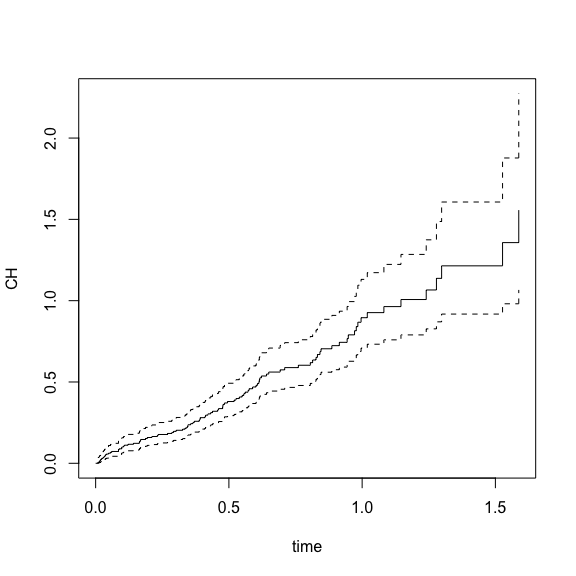We can further play with the procedure by adjusting the coverage level and also truncating the data:

opt_H <- optband::opt.ci(S, conf.level = 0.90, fun = "cumhaz", tl = .1, tu = .9)
plot(opt_H, fun="cumhaz", xlab="time", ylab="CH", mark.time=FALSE)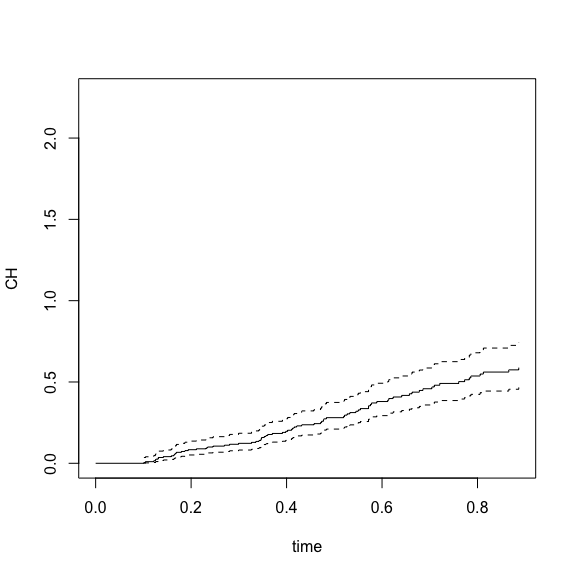And compare the results of this band to the Equal Precision and Hall-Wellner bands (for this we will use the km.ci package):

color <- c("grey", "darkblue", "red", "green")
plot(S, mark.time=FALSE, xlab="time", ylab="KM", col = "white")

lines(opt_S, col=color, lty=2, lwd=2, mark.time=F)
e <- km.ci::km.ci(S, conf.level = 0.95, method = "epband")
h <- km.ci::km.ci(S, conf.level = 0.95, method = "hall-wellner")
lines(e, col=color, lty=3, lwd=2, mark.time=F)
lines(h, col=color, lty=4, lwd=2, mark.time=F)
lines(S,col="grey", lwd=2, mark.time=F)

legend("topright", c("KM", "optband", "epband", "hall-wellner"),
lwd=2, lty=1:4, col=color)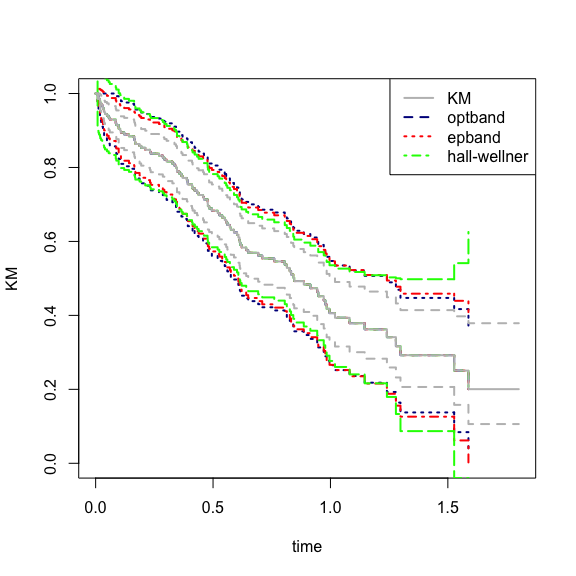Lastly, while the 2-sample survival function difference confidence bands are intractable with this method, we can estimate confidence bands for the cumulative hazard function difference. For this, we’ll use the bladder data set from the survival package, first subsetting upon only the times until first recurrence:

dat <- bladder[bladder$enum==1,] Hdif = survival::survfit(Surv(stop, event) ~ rx, type="kaplan-meier", data=dat) opt_Hdif <- optband::opt.ci(Hdif, fun="cumhaz", conf.level = 0.95, samples=2) plot(opt_Hdif$difference~opt_Hdif$time, xlab="t", ylim=c(-1.5,1), main=expression(hat(Lambda)(t)-hat(Lambda)(t)), ylab="", col = "white") lines(opt_Hdif$difference~opt_Hdif$time, col = "grey", lty=1, lwd=2) lines(-log(opt_Hdif$upper)~opt_Hdif$time, col=4, lty=3, lwd=2) lines(-log(opt_Hdif$lower)~opt_Hdif\$time, col=4, lty=3, lwd=2)